Editor Ratings:
User Ratings:
[Total: 0 Average: 0]

Matrix Reckoner is a free Matrix calculator for Windows which lets you solve Matrix problems. The freeware makes solving matrix problems as easy as ABC. All you need is to enter the matrix problem and click the Calculate option to get the solution instantly. The freeware supports matrix problems of up to order 6. You can use it solve any matrix problems which has a maximum order 6.

The mathematical operations which you can perform on matrix are Addition, Multiplication, and Subtraction. Apart from this, you can also use this freeware to calculate the Inverse, Transpose, and Determinant of any matrix with just one click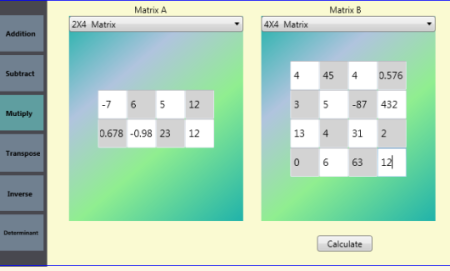## Using this Free Matrix Calculator for Windows:

You need to download and run the executable file of Matrix Reckoner. As I said that its executable file, it means you don’t have install it and you only have to double click on it to launch the freeware. When the freeware is launched, you will see the interface shown below.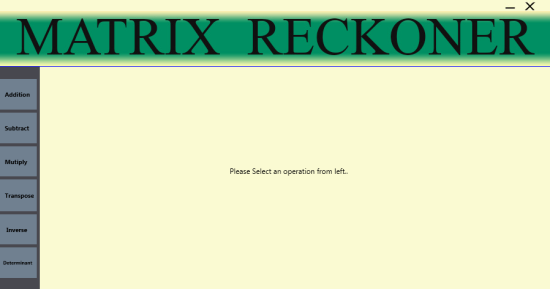Now for performing mathematical operations, choose the operation (Addition, Multiplication, or Subtraction) from the option on the left sidebar. When you have selected the operation, choose the order of Matrix which you want to solve.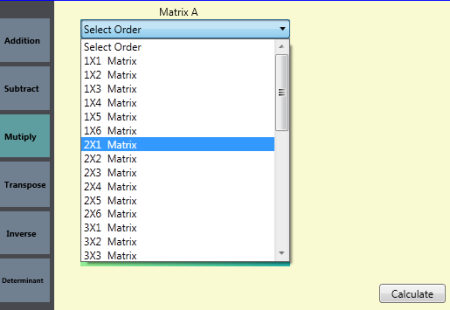After selecting the left-hand side matrix, you have to choose the order of right-hand matrix. On selecting both matrix, you have to enter the value of each cell. You can enter the numbers easily by using your keyboard. You can enter any number be it integer or decimal. The app supports both positive and negative integer.When you have entered both the matrices, just click on the Calculate option to get the answer. The answer is shown to you on the right side instantly.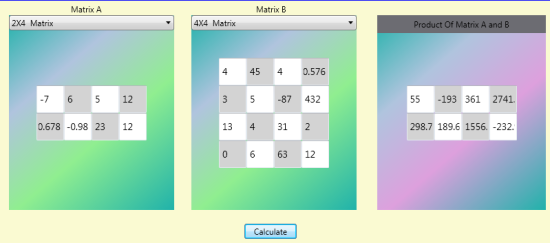Similarly for calculating Inverse, Transpose or the Determinant, you only have to click on that particular option. After this, choose the order of matrix, enter the numerical values using keyboard and click on Calculate to get the answers.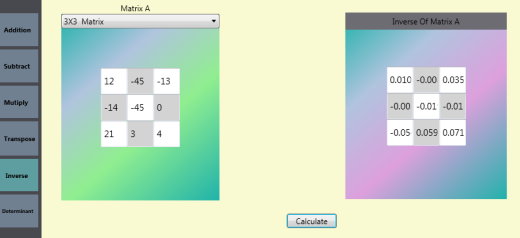You may also like: 14 Best Free Math Software, Matrix Determinant.

## Final Verdict:

Matrix Reckoner is a good Matrix calculator for Windows which is extremely easy to use. You just choose the type of problem, order of matrix, enter values and get the answer. The only feature I missed is that it doesn’t show complete step by step solution. It’s good for those who just want to know the solution of the problem.